# Classifying scalene, isosceles, and equilateral triangles by side lengths or angles Online Quiz

Following quiz provides Multiple Choice Questions (MCQs) related to Classifying scalene, isosceles, and equilateral triangles by side lengths or angles. You will have to read all the given answers and click over the correct answer. If you are not sure about the answer then you can check the answer using Show Answer button. You can use Next Quiz button to check new set of questions in the quiz.Q 1 - Identify the given triangle as an equilateral, isosceles or a scalene triangle.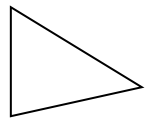### Explanation

Step 1:

Given triangle has sides of different lengths.

Step 2:

So given triangle is a scalene triangle.

Q 2 - Identify the given triangle as an equilateral, isosceles or a scalene triangle.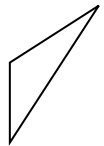### Explanation

Step 1:

Given triangle has sides of different lengths.

Step 2:

So given triangle is a scalene triangle.

Q 3 - Identify the given triangle as an equilateral, isosceles or a scalene triangle.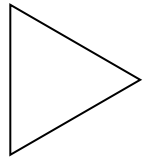### Explanation

Step 1:

Given triangle has all sides of same length.

Step 2:

So given triangle is an equilateral triangle.

Q 4 - Identify the given triangle as an equilateral, isosceles or a scalene triangle.### Explanation

Step 1:

Given triangle has sides of different lengths.

Step 2:

So given triangle is a scalene triangle.

Q 5 - Identify the given triangle as an equilateral, isosceles or a scalene triangle.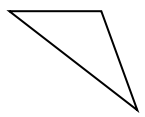### Explanation

Step 1:

Given triangle has sides of different lengths.

Step 2:

So given triangle is a scalene triangle.

Q 6 - Identify the given triangle as an equilateral, isosceles or a scalene triangle.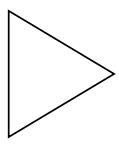### Explanation

Step 1:

Given triangle has all sides of same length.

Step 2:

So given triangle is an equilateral triangle.

Q 7 - Identify the given triangle as an equilateral, isosceles or a scalene triangle.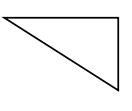### Explanation

Step 1:

Given triangle has sides of different lengths.

Step 2:

So given triangle is a scalene triangle.

Q 8 - Identify the given triangle as an equilateral, isosceles or a scalene triangle.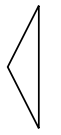### Explanation

Step 1:

Given triangle has two sides of same length.

Step 2:

So given triangle is an isosceles triangle.

Q 9 - Identify the given triangle as an equilateral, isosceles or a scalene triangle.### Explanation

Step 1:

Given triangle has two sides of same length.

Step 2:

So given triangle is an isosceles triangle.

Q 10 - Identify the given triangle as an equilateral, isosceles or a scalene triangle.### Explanation

Step 1:

Given triangle has sides of different lengths.

Step 2:

So given triangle is a scalene triangle.

classifying_scalene_isosceles_and_equilateral_triangles_by_side_lengths_or_angles.htm